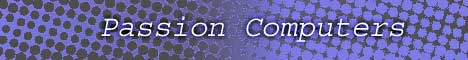Home  | Computers  | Networking  | Programming  | Electronics  | How to   | Help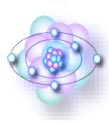Jump to Related The ATOM OHMs' law

 Overview
To understand most of electronics you must first understand what all makes it work, for discussion you will lean about current, voltage, power and how you may be able to find and apply these values.
First of all you need to know what makes up electronics, as you might have guessed it is the electron and how you manipulate how they travel through conductors. As you start learning electronics you will start to realize that this whole field is quite complicated and almost disturbing but as you learn more you will also start to realize that it is based upon simple physics. You might look at the photo resistor and wonder what kind of satanic voodoo magic is going on but it turns out that it is quite simple if you know how electronics works.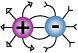For now lets look at the electron, and as we know from discussions like "The Atom", you learned that opposite charges are attracted to each other and similar charges repel each other.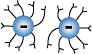As you have or will learn in discussions like "Conductor Vs Insulator", that conductors allow electrons to flow in a direction that you want it and insulators do not allow electrons to flow. This is the concept behind the wire, the metal core or conductor is responsible for transporting electrons from one point to the next with the insulator prevents electrons from straying off to another insulator or metal object.
However, in most cases that interact with the world you need a great amount of electrons.
 Current
Current is defined as the flow of electrons through a given point. It is somewhat of a mystery for many as to how it actually works. You must think of current as groups of electrons that are constantly trying to reach a state of equilibrium.

Lets use the battery as an example because there is some confusion as to how it works. Some believe that there are two charges that come out of the battery and electrons flow at a slow speed are completely wrong. The physics of the battery and current flow is much simpler if you know how the atom works.
You know form chapters like "the atom" that electrons are constantly trying to reach the nucleus but the electrons are spinning so fast that they are forced outward. Just like if you spun you arm in circles as fast as you could your blood would rush to your fingertips.
You also learned that protons at the center of the atom are secured to the neutrons that make up the nucleus. The number of protons within the nucleus determine how many electrons the atom can hold, the more the protons the more electrons with a factor of 1.1.

Take the silicon atom for example, it has 14 protons and 14 electrons in a constant state of balance.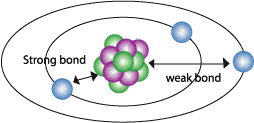What makes a good conductor is a material with 7 or more electrons, this means that there is s third shell meaning that the attraction between the outer shell and the nucleus is weaker. This means that the outer atoms can be taken away easily or the atom can gain electrons if the nucleus permits it.

What we can now determine is that protons do not travel through wires, rather they are a source of pull. The negative terminal of the battery is considered to have a negative charge because there are more negatively charged electrons are crammed into that terminal. The positive side of the battery has had many electrons stripped off the atoms, this creates an electron void with more protons then electrons which is like a vacuum for electrons. The thirst of the atoms on the positive side for electrons and the pressure for the excess electrons on the negative side creates Electromotive Force (EMF). Lets look at the work Electromotive Force, Electro meaning electron, Motive meaning motion, and Force meaning pressure or VOLTAGE. So when there is a void or excess source of electrons there will be a voltage present, ready to do work.
 The physics behind current flow is not that the electron magically wants to travel to travel form one point to another but it is the pressure is force upon other electrons when an electron is introduced. It is also important that you know that a conductor "wire" already contains many electrons with in it but when another electron is introduced, all the proceeding electrons are forced to squeeze together. This creates a voltage that pushes on the next electron and that electron does it to the next creating current flow. The concept of voltage will be explained in a minute but lets understand first the mathematical symbols and unit of current.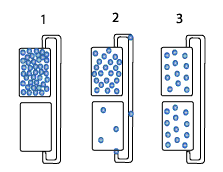Symbols & Measurements
 Current is measured in the Amp and the symbol is the uppercase A, this is a large number of electrons and is why you more often the not be measuring in miliA and µA.    In many cases like power supplies and stereo amplifiers you may experience tens or even thousands of amps, this is where you leave the engineering and construction to the pro's.    How big is an amp?    The number of electrons flowing in a circuit of one amp is equivalent to 6.28 billion electrons flowing across any given point. Although current is what kills people and burns out your circuits that you worked so hard on, voltage is what makes current possible.
 Voltage
 Voltage is known as the force that moves electrons along in a given direction.    To demonstrate how voltage works lets take static electricity, static electricity is of little current but the voltage is in the 2500. This gives the current the ability to travel through the air you breath and penetrate your skin. It only gives you a little shock but when you take electronics into consideration where computer chips operate with 5 volts. You probably can already guess it, what is going to happen when you touch the circuit with 2500 volts is that you are going to fry a circuit that only takes 250V to destroy the whole computer chip "processor". So as you can see there are definite characteristics with current and voltage, current is the flow of electrons which does all the work and damage while voltage is the pressure of current. The greater the pressure "voltage" a circuit has the greater the force of behind electron flow "current".    It should be noted that if voltage was absent from a circuit then the electrons would be stagnant and would not move due to a lack of force. Likewise of current was not present then there would be no use or interest for it because current is electron flow.
 Power
 Calculator    Power takes Electromotive Force (Voltage), current (Amps), and Time (seconds), to determine how many electrons are flowing through a given point in one second. The unit for measuring power is what watt, and the formula for finding the total watts within a circuit is as follows:    Let's say that you have 3 volts, with a current of µ25A, in a period of 1 second. The power traveling through the circuit can be found using the following equation:    Power = (Volts * current) / seconds    3V * 0.000025 = 0.000075A    .000075A / 1 second(s) = .000075A or 75 µW
 How to use it
 Now there can be different levels of current with different levels of voltage but still exert the same amount of force. There however comes a point to where there can be too much voltage and too little current to perform tasks. Also there can be too much current and too little voltage to do any work that us useful.    So you must keep in mind of what you your main objective is before you start to make power supplies or any other circuit for that matter.
 Page Home

Home | Contact us | Our History | Link to us | Relations
A Passion Production ©opyright 2000